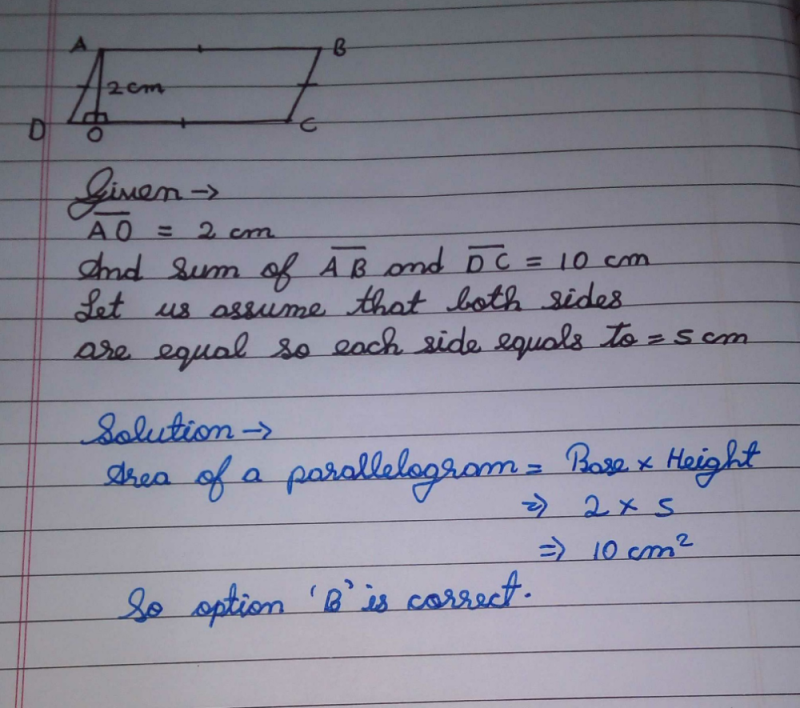Courses

# If the parallel sides of a parallelogram are 2 cm apart and their sum is 10 cm then its area is:a)20 cm2b)10 cm2c)5 cm2d)none of theseCorrect answer is option 'B'. Can you explain this answer? Related Test: Mensuration - MCQ

## Class 8 Question

By Avneet Kaur · Jan 03, 2020 ·Class 8
Akshat Bhatt answered Jan 03, 2020Charming Rocky answered Jan 03, 2020
Ar.=(1/2)×10×2.. ..

Distance between the two parallel lines = h =2 cm
sum of length of sides =10cm
As we know the sides of parallelogram are equal
let the length of side be 'b' =base of parallelogram
2b =10
b = 5 cm
area of parallelogram = h×b
= 2×5
=10 cm2